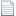# 求偏微分方程的数值解（有限差分法、配置法、Galerkin方法）pde_approximation.zipFinite difference method

• approximate differential equations using finite difference equations to approximate derivatives

Collocation method

• uses a finite-dimensional space of basis functions and collocation points to approximate PDEs

Galerkin's technique

• uses orthogonality of a set of basis function to turn PDEs into coupled sets of ODEs

Finite difference method

• The derivatives of the PDE are approximated by linear combinations of function values at the structured grid points, using a Taylor series expansionCollocation method

• Define the basis function (usually polynomials) and approximate solution:• Calculate residual function by substituting the candidate function into the original PDE

• Choose the collocation points at which the candidate function must exactly matchGalerkin's method

• Define the basis function (usually orthogonal polynomials) and approximate solution as• Calculate residual function by substituting the candidate function into the original PDE

• Minimize the residual using Least Squares ErrorMaple中的计算文件：Whitepaper-Developing_Mathematical_Models_of_Batteries.pdf

http://wap.sciencenet.cn/blog-516836-1292219.html

## 全部精选博文导读

GMT+8, 2021-12-1 11:36